# Lie triple system

A triple system is a vector spaceover a fieldtogether with a-trilinear mapping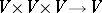.

A vector spacewith triple product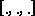is said to be a Lie triple system if(a1)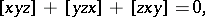(a2)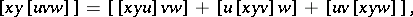(a3)

for all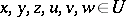.

Setting, then (a3) means that the left endomorphism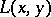is a derivation of(cf. also Derivation in a ring). Thus one denotesby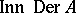.

Letbe a Lie triple system and let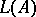be the vector space of the direct sum ofand. Thenis a Z2-graded Lie algebra with respect to the product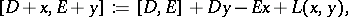where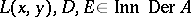,.

This algebra is called the standard embedding Lie algebra associated with the Lie triple system. This implies thatis a homogeneous symmetric space (cf. also Homogeneous space; Symmetric space), that is, it is important in the correspondence with geometric phenomena and algebraic systems. The relationship between Riemannian globally symmetric spaces and Lie triple systems is given in [a4], and the relationship between totally geodesic submanifolds and Lie triple systems is given in [a1]. A general consideration of supertriple systems is given in [a2] and [a5].

Note that this kind of triple system is completely different from the combinatorial one of, e.g., a Steiner triple system.

How to Cite This Entry:
Lie triple system. Encyclopedia of Mathematics. URL: http://encyclopediaofmath.org/index.php?title=Lie_triple_system&oldid=43183
This article was adapted from an original article by Noriaki Kamiya (originator), which appeared in Encyclopedia of Mathematics - ISBN 1402006098. See original article